Electrical Dost

# Derive the expression for energy stored in a magnetic field.The energy required to establish a magnetic field gets stored in it as potential energy. This energy can be recovered when the magnetic field established gets collapsed.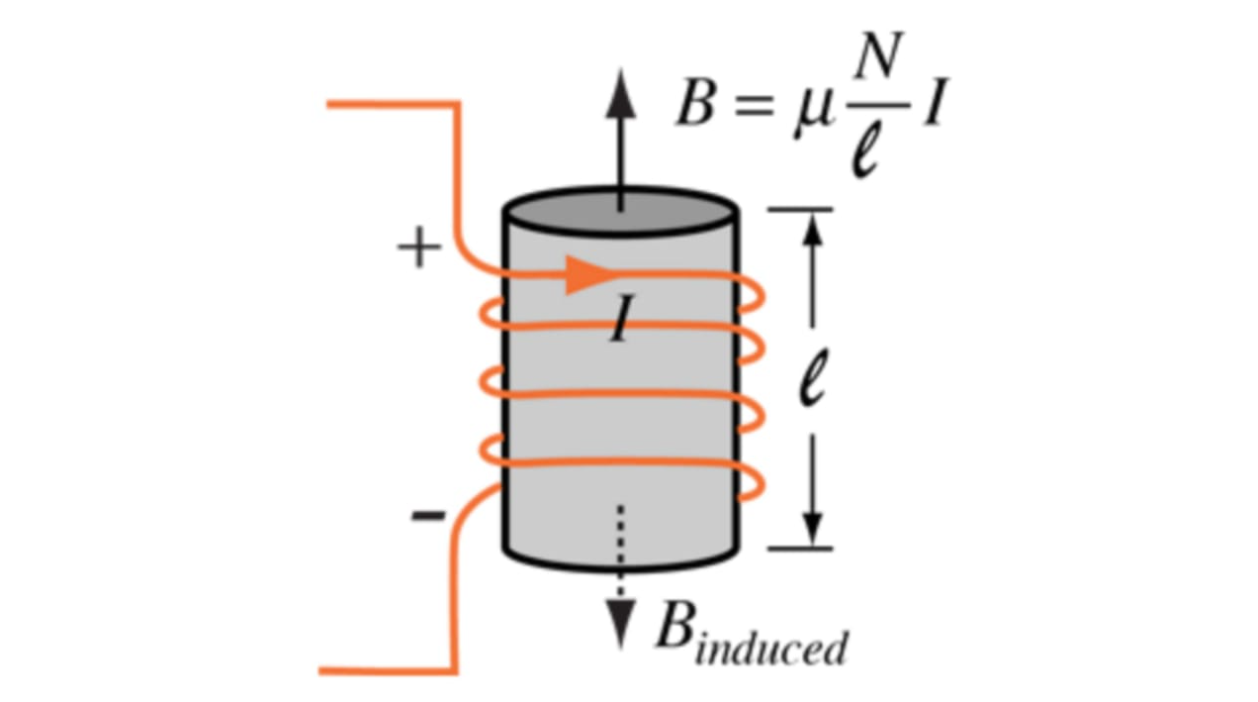Consider a solenoid, the current through which can be controlled with the help of switch S, resistance R.

Initially, switch ‘S’ is open, so current through coil, I is zero. When switch is closed, current will try to build its value equal to I. neglect the resistance of the coil. It will take some time to increase the current from ‘zero’ to ‘I’ say ‘dt’ seconds. Hence e.m.f. will be induced in coil.

Let induced e.m.f. in a coil be ,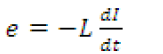This opposes a supply voltage. So supply voltage ‘V’ supplies energy to overcome this, which ultimately gets stored in magnetic field.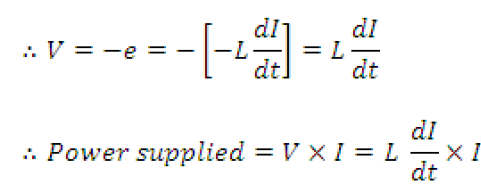∴Energy supplied in time dt is,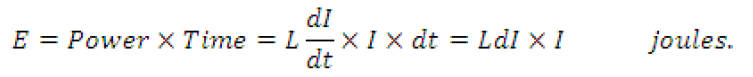This is energy supplied for change in current of dI but actually current changes from zero to I.

∴ Integrating above, total energy stored is,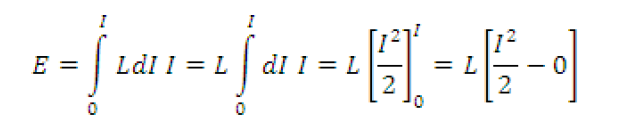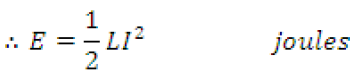### Krushna Bochare

Electrical Engineer, Content Creator, Working At PowerIT Solutions Pvt Ltd.

## Related Posts

### Types of Capacitors

There are three basic types of Capacitors given in the below: (1) Parallel plate capacitor (2) Cylindrical capacitor: (3) Spherical capacitor: Let’s discuss in details